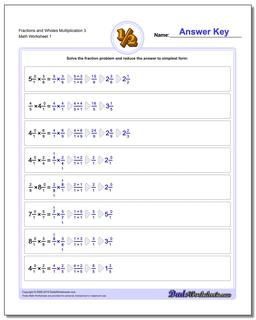# Math Worksheets: Fraction Multiplication: Fraction Multiplication: Fractions and Wholes Multiplication 3## Fractions and Wholes Multiplication 3

PropertyValue
DescriptionFractions and Wholes Multiplication 3: Basic multiplying fractions against wholes. Each problem on these worksheets has a single fraction multiplied by a whole value.
Resource TypeWorksheet